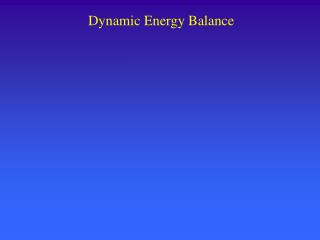Download PresentationDynamic Energy Balance

# Dynamic Energy Balance

Download Presentation## Dynamic Energy Balance

- - - - - - - - - - - - - - - - - - - - - - - - - - - E N D - - - - - - - - - - - - - - - - - - - - - - - - - - -
##### Presentation Transcript

1. Dynamic Energy Balance

2. rxn # Dynamic Energy Balance • Last time: well-mixed CSTR w/flow & reaction • for multiple reactions:

3. Simplifications For moderate T changes use average Cpi  assume Cpi are constant • ACC Term:

4. Simplifications • Also: • Simplified Form: well-mixed CSTR w/flow, reaction & avg. Cp

5. vo To Steam in Ts v T  Condensate In-Class Exercise Liquid flows continuously into an initially empty tank, which contains a full-depth heating coil. As the tank fills, an increasing proportion of the coil is covered by liquid. Once the tank is full, the liquid starts to overflow into the discharge pipe, but heating is maintained. How long does it take the system to reach steady state, what is the final exit temperature, and how long does it take before the tank “overflows”?

6. In-Class Exercise

7. In-Class Exercise

8. In-Class Exercise

9. In-Class Exercise

10. In-Class Exercise

11. Energy Balance on Vessel Jacket • Assumptions: • jacket well insulated • liquid in jacket is well mixed • Vj(Volume of jacket fluid) is constant • j is constant • no work nor reaction in jacket • If Constant Tj: assume j1 = j2 Tj2 = constant • Jacket energy balance:

12. Energy Balance on Vessel Jacket(especially if Tj varies significantly) • If Tj not constant: (for small systems, low flow, or not well mixed)  assume j1 = j2 i.e. constant 

13. Energy Balance on Vessel Jacket(Tj not constant) • Energy balance on heat transferred:  Rate of energy transfer from jacket to the reactor = Rate of energy loss by jacket fluid  from  then into 

14. Kj Energy Balance on Vessel Jacket(Tj not constant)

15. j Effect of Flow on Kj so as j  Kj  ??

16. Energy Balance on Vessel Jacket(Tj not constant) • Assumptions: • jacket well insulated • liquid in jacket is more like plug flow (i.e cooling coil) • Vj(Volume of jacket fluid) is constant • j is constant • no work nor reaction in jacket • Energy balance on jacket: and:

17. Small Vessel Jackets • For small vessels or high pressure systems the thickness of the vessel wall can be significant…thus one needs to consider the thermal capacity of the wall. • Energy balance on wall: • Energy balance for jacket: Tj constant

18. In-Class Exercise Liquid flows continuously into continuous stirred tank reactor, which is fully-jacketed and well-mixed. At a certain time, reactant A is introduced into the feed liquid, such that the volumetric flowrate remains constant. 1) Show that the steady state solution to the problem gives a reactor T of ~331 K. 2) With no control (Kc=0), use the dynamic model to find the SS solution. 3) Add proportional control to the dynamic model. Examine the effect of varying Tset from 300 - 375 K, and Kc from -1 to 5. 4) Add integral control to the controller equation. What effect does this have? TC o, To, Cao TE  T j, Tj  V, T Ca, Cb jo, Tjo

19. In-Class Exercise • From before: • Let: • So:

20. In-Class Exercise with: Kc = controller gain • PI controller: • P only controller: with: I = integral time • Let: or: • Then: initial condition?

21. In-Class Exercise

22. In-Class Exercise

23. In-Class Exercise

24. In-Class Exercise

25. In-Class Exercise : SS Solution

26. In-Class Exercise: SS Solution

27. In-Class Exercise: dynamic - SS

28. In-Class Exercise : dynamic - SS

29. In-Class Exercise

30. In-Class Exercise